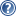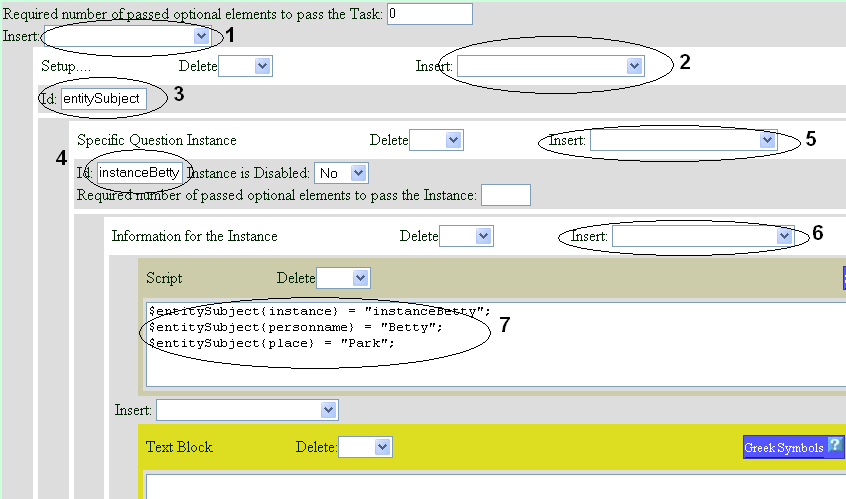###LON-CAPA Help

To create difference versions of Bridge Tasks for each student, parts of the questions are defined using variables. Each variable contains multiple instances of possible values and LON-CAPA randomly selects an instance to give to the student. Figureshows a screenshot of this process.Figure 1: Bridge Task variable creation screenshot

To create a variable:

1. Choose "Setup ..." in the insert pull-down menu (circled 1 in figure).

2. Press the Submit Changes and Edit just above the work space.

3. A new box should appear with the label "Setup ...". In this box, fill out the id box (circled 3) with any text that is unique to unique to the document. This id is the name of the variable and will be used when creating the values for the variable.

4. The setup box has an insert pull-down menu next to the label (circled 2), select "Specific Question Instance" in this pull down menu, then again press the Submit Changes and Edit button.

5. This creates one single instance of a set of possible values. For each instance created, a new "Specific Question Instance" must be created.

6. Right now a box should appear inside the "Setup... " box with the label "Specific Question Instance". Insert a unique id for that instance which can be any unique text (circled 4). This id is the instance name and is used as one of the property of the variable.

7. In the question instance block, select "Information for the Instance" in the insert pull down (circled 5). Again press submit button.

8. Add a new script (circled 6) in the insert pull down. A new text block should appear.

9. In this text box, a perl script will be created (circled 7). A set of parameters for this variable is added. The syntax to set the parameter of a variable is '\$variableName {fieldname} = "fieldValue"'. The variable name is taken from the id field of the Setup block, thefield name is the name of the parameter the author sets, and the fieldValue is simply the value of the field. The first parameter that must be set is the instance field, with the value being an identifier of the instance (which is the id of the specific question instance block).

The example below shows two instances of this script for the variable entitySubject with two instances, 'instanceHarry' and 'instanceBetty'.

The first instance (instanceHarry) is:

```	\$entitySubject{instance} = "instanceHarry";
\$entitySubject{personname} = "Harry";
\$entitySubject{place} = "zoo";

```

The second instance (instanceBetty) is:

```	\$entitySubject{instance} = "instanceBetty";
\$entitySubject{personname} = "Betty";
\$entitySubject{place} = "park";

```

The example above describes a variable question. It has two different possible values for the entity "subject", Harry and zoo or Betty and park. Variables can be placed inside the questions by using the variable name and field name. The example of the usage of this variable inside the question is this text:

This is a test question. \$entitySubject{personname} went to the \$entitySubject{place}. .

The LON-CAPA engine will replace any instance of \$ < variable_name > ( < property_name > ) with the correct value, depending on the randomly chosen instance.

Based on this code, two different questions are possible:

1. This is a test question. Harry went to the zoo

2. This is a test question. Betty went to the park